# Case Study Free Variables CS 5010 Program Design

• Slides: 17
Download presentation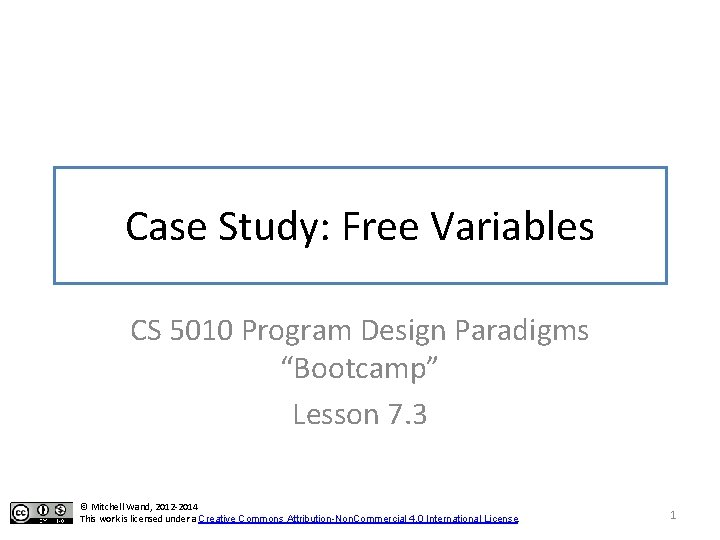Case Study: Free Variables CS 5010 Program Design Paradigms “Bootcamp” Lesson 7. 3 © Mitchell Wand, 2012 -2014 This work is licensed under a Creative Commons Attribution-Non. Commercial 4. 0 International License. 1Learning Objectives • At the end of this lesson the student should be able to: – explain the notion of a free variable – identify the free variables in the expressions of a simple programming language – explain two algorithms for finding the free variables of an expression in a simple programming language 2A Tiny Programming Language: Fred • The Information: Fred. Exp = Variable | (lambda (Variable) Fred. Exp) | (Fred. Exp) Variable = x | y | z |. . . | xx | yy | zz |. . . The setting is that we are writing a compiler for a tiny programming language, called Fred. Here is some information about expressions in Fred: A Fred-expression is either a variable, or a lambda expression, or an application. We've written down some suggestive notation here, but we're not specifying exactly how these expressions are going to be written down; we're only saying what kind of expressions there and what they might (repeat, might) look like. 3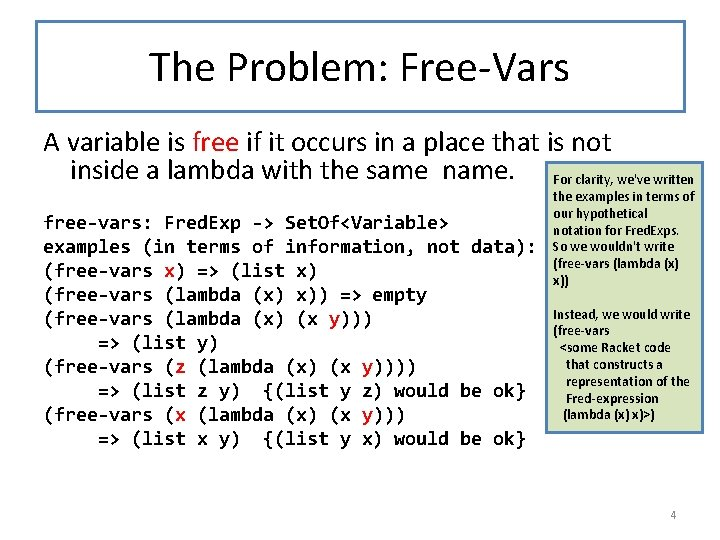The Problem: Free-Vars A variable is free if it occurs in a place that is not inside a lambda with the same name. For clarity, we've written free-vars: Fred. Exp -> Set. Of<Variable> examples (in terms of information, not data): (free-vars x) => (list x) (free-vars (lambda (x) x)) => empty (free-vars (lambda (x) (x y))) => (list y) (free-vars (z (lambda (x) (x y)))) => (list z y) {(list y z) would be ok} (free-vars (x (lambda (x) (x y))) => (list x y) {(list y x) would be ok} the examples in terms of our hypothetical notation for Fred. Exps. So we wouldn't write (free-vars (lambda (x) x)) Instead, we would write (free-vars <some Racket code that constructs a representation of the Fred-expression (lambda (x) x)>) 4Data Design (define-struct var (name)) (define-struct lam (var body)) (define-struct app (fn arg)) ; ; ; ; We will represent Fred. Exps as recursive structures. This is our firstchoice representation for information in Racket— you can almost never go wrong choosing that representation. A Fred. Exp is one of (make-var Symbol) (make-lam Symbol Fred. Exp) (make-app Fred. Exp) INTERPRETATION: the cases represent variables, lambdas, and applications, repectively. 5Symbols and Quotation • • Our data design uses symbols. A Symbol is a primitive data type in Racket. It looks like a variable. To introduce a symbol in a piece of code, we precede it with a quote mark. For example, 'z is a Racket expression whose value is the symbol z. 6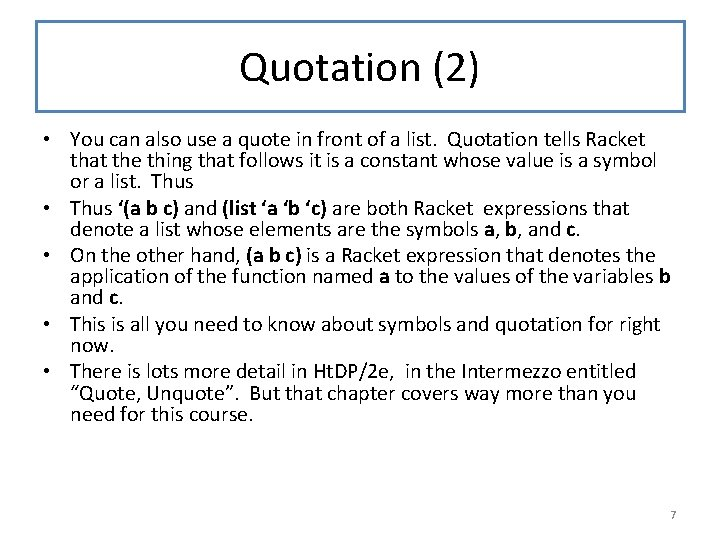Quotation (2) • You can also use a quote in front of a list. Quotation tells Racket that the thing that follows it is a constant whose value is a symbol or a list. Thus • Thus ‘(a b c) and (list ‘a ‘b ‘c) are both Racket expressions that denote a list whose elements are the symbols a, b, and c. • On the other hand, (a b c) is a Racket expression that denotes the application of the function named a to the values of the variables b and c. • This is all you need to know about symbols and quotation for right now. • There is lots more detail in Ht. DP/2 e, in the Intermezzo entitled “Quote, Unquote”. But that chapter covers way more than you need for this course. 7Data Design (2) EXAMPLE: (z (lambda (x) (x y))) is represented by (make-app (make-var 'z) (make-lam 'x (make-app (make-var 'x) (make-var 'y)))) Now that we’ve briefly explained about symbols and quotation, we can give an example of the representation of a Fredexpression. 8Destructor Template In Racket, #; marks the next S-expression as a comment. So this definition is actually a comment. This is handy for templates. ; ; fredexp-fn : Fred. Exp -> ? #; (define (fredexp-fn f) (cond [(var? f) (. . . (var-name f))] [(lam? f) (. . . (lam-var f) (fredexp-fn (lam-body f)))] [(app? f) (. . . (fredexp-fn (app-fn f)) (fredexp-fn (app-arg f)))])) 9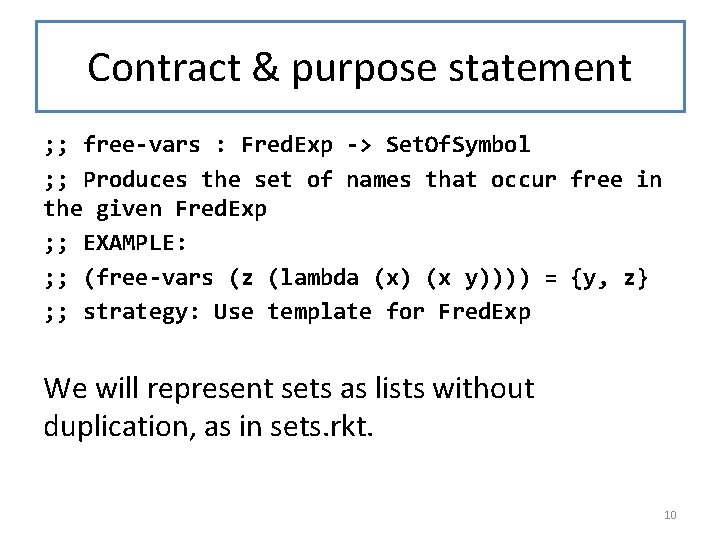Contract & purpose statement ; ; free-vars : Fred. Exp -> Set. Of. Symbol ; ; Produces the set of names that occur free in the given Fred. Exp ; ; EXAMPLE: ; ; (free-vars (z (lambda (x) (x y)))) = {y, z} ; ; strategy: Use template for Fred. Exp We will represent sets as lists without duplication, as in sets. rkt. 10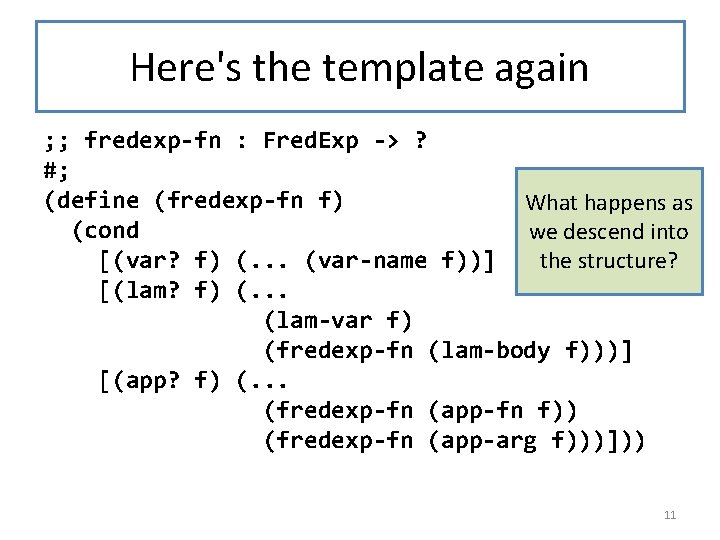Here's the template again ; ; fredexp-fn : Fred. Exp -> ? #; (define (fredexp-fn f) What happens as (cond we descend into the structure? [(var? f) (. . . (var-name f))] [(lam? f) (. . . (lam-var f) (fredexp-fn (lam-body f)))] [(app? f) (. . . (fredexp-fn (app-fn f)) (fredexp-fn (app-arg f)))])) 11What happens as we descend into the structure? • We lose information about which lambdavariables are above us. • So we'll add a context variable to keep track of the lambda-variables above us – when we hit a variable, see if it's already in this list. If so, it's not free in the whole expression. – This is like the counter in mark-depth 12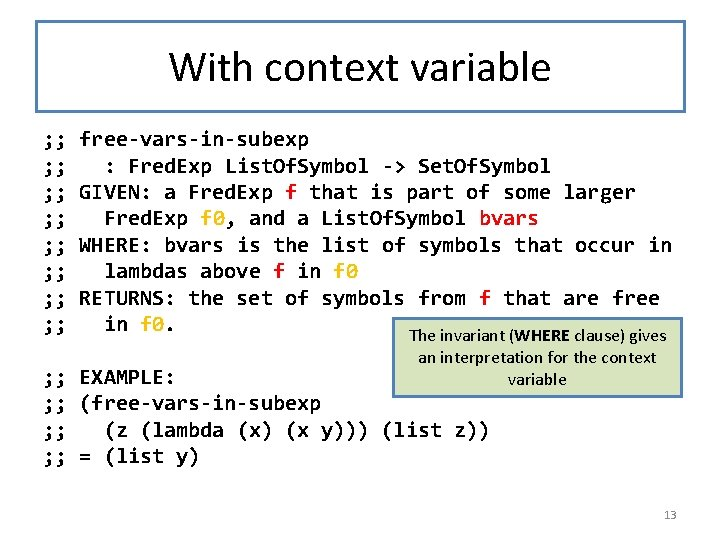With context variable ; ; ; ; free-vars-in-subexp : Fred. Exp List. Of. Symbol -> Set. Of. Symbol GIVEN: a Fred. Exp f that is part of some larger Fred. Exp f 0, and a List. Of. Symbol bvars WHERE: bvars is the list of symbols that occur in lambdas above f in f 0 RETURNS: the set of symbols from f that are free in f 0. The invariant (WHERE clause) gives an interpretation for the context variable ; ; EXAMPLE: ; ; (free-vars-in-subexp ; ; (z (lambda (x) (x y))) (list z)) ; ; = (list y) 13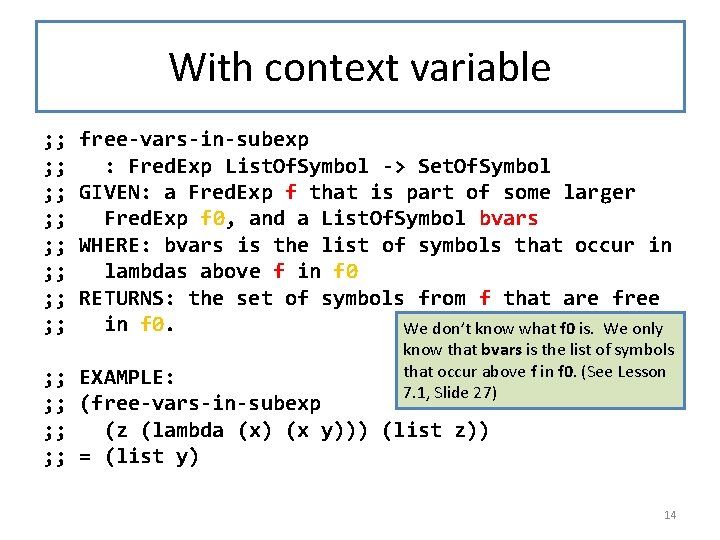With context variable ; ; ; ; free-vars-in-subexp : Fred. Exp List. Of. Symbol -> Set. Of. Symbol GIVEN: a Fred. Exp f that is part of some larger Fred. Exp f 0, and a List. Of. Symbol bvars WHERE: bvars is the list of symbols that occur in lambdas above f in f 0 RETURNS: the set of symbols from f that are free in f 0. We don’t know what f 0 is. We only know that bvars is the list of symbols that occur above f in f 0. (See Lesson 7. 1, Slide 27) ; ; EXAMPLE: ; ; (free-vars-in-subexp ; ; (z (lambda (x) (x y))) (list z)) ; ; = (list y) 14Function Definition Is the variable already bound? ; ; STRATEGY: Struct Decomp on f : Fred. Exp (define (free-vars-in-subexp f bvars) (cond [(var? f) (if (my-member? (var-name f) bvars) empty (list (var-name f)))] [(lam? f) (free-vars-in-subexp (lam-body f) (cons (lam-var f) bvars))] [(app? f) (set-union (free-vars-in-subexp (app-fn f) bvars) (free-vars-in-subexp (app-arg f) bvars))])) Adds the lambda-variable to the list of bound variables in the body, so the called function's WHERE clause will become true. 15Function Definition (part 2) ; ; free-vars : Fred. Exp -> Set. Of. Symbol ; ; Produces the set of names that occur free in the given Fred. Exp ; ; EXAMPLE: ; ; (free-vars (z (lambda (x) (x y)))) ; ; = {y, z} There are no variables bound above the top. ; ; Strategy: call a more general function (define (free-vars f) (free-vars-in-subexp f empty)) 16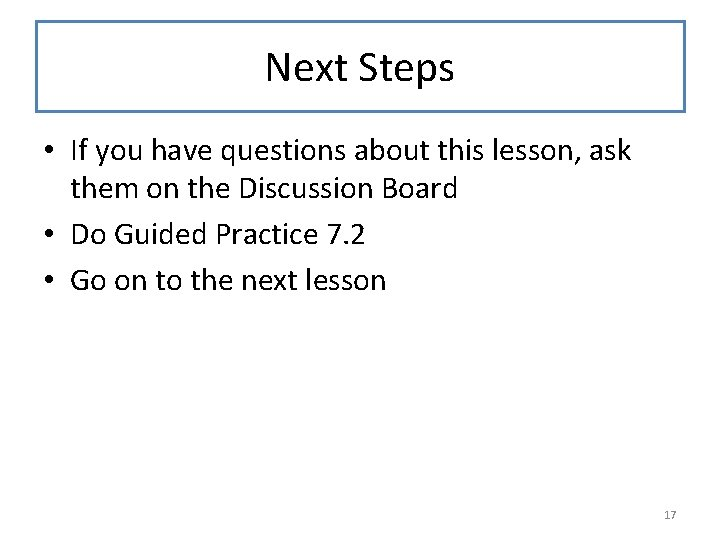Next Steps • If you have questions about this lesson, ask them on the Discussion Board • Do Guided Practice 7. 2 • Go on to the next lesson 17